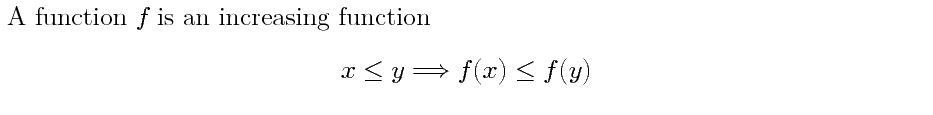# Comibined characters in Math

Posted on October 15, 2017

There is a new feature in ConTeXt that replaces some ascii math (I am using this term informally. The symbols do not match the asciimath symbol list). It is enabled using1

\setupmathematics[collapsing=3]


and replaces a combination of characters by a glyph. For example,

\setupmathematics[collapsing=3]
A function $f$ is an increasing function
\startformula
x <= y  ===> f(x) <= f(y)
\stopformula


givesNotice that <= got translated to ≤ (\le) and ===> got translated to ⟹ (\Longrightarrow).

ConTeXt version 2017.08.15 or newer is needed for these mappings to work. Here is the complete list of comining characters that are available:

shortcut Mapped to
'' \doubleprime
''' \tripleprime
'''' \quadprime
:: \squaredots
:= \colonequals
=: \equalscolon
::= \coloncolonequals
-: \minuscolon
/< \nless
/> \ngtr
<= \le
/<= \nleq
>= \ge
/>= \ngeq
/= \neq
=> \eqgtr
=< \eqless
<=> \lesseqqgtr
>=< \gtreqqless

<< \ll
’>> \gg
<<< \lll
>>> \ggg
== \equiv
/== \nequiv
=== \eqequiv (a virtual character for mapping purposes)
-> \rightarrow
<- \leftarrow
<-> \leftrightarrow
--> \longleftarrow
<-- \longrightarrow
<--> \longleftrightarrow
==> \Rightarrow
<== \Leftarrow
<==> \Leftrightarrow
===> \Longrightarrow
<=== \Longleftarrow
<===> \Longleftrightarrow
|| \doubleverticalbar
||| \tripleverticalbar

If the font does not contain the appropriate glyph, then the above will silently fail (e.g., Latin Modern Math does not have ::=). If something goes wrong, partial debug information is available using:

\enabletrackers[math.collapsing]


This shows the following tracking information on the console (and also writes it to the log file)

mathematics     > collapsing > enabling math collapsing
mathematics     > collapsing > creating ligature ″ (U+02033) from specials
mathematics     > collapsing > creating ligature ‴ (U+02034) from specials
mathematics     > collapsing > creating ligature ⁗ (U+02057) from specials
mathematics     > collapsing > creating ligature ∷ (U+02237) from mathlist
mathematics     > collapsing > creating ligature ≔ (U+02254) from mathlist
mathematics     > collapsing > creating ligature ≕ (U+02255) from mathlist
mathematics     > collapsing > creating ligature ⩴ (U+02A74) from specials
...

Notice that there are two mechanisms for collapsing mathlist and specials (I don’t quite understand the difference except that the specials mechanism is old and related for some font stuff while the mathlist mechanism is new). The relevant information is available in char-def.lua. For example, for ::= (which is a special), the entry in char-def.lua is

[0x2A74]={
category="sm",
description="DOUBLE COLON EQUAL",
direction="on",
linebreak="al",
mathclass="relation",
mathname="coloncolonequals",
specials={ "compat", 0x3A, 0x3A, 0x3D },
unicodeslot=0x2A74,
},


On the other hand, for :: (which is a mathlist), the entry in char-def.lua is:

[0x2237]={
category="sm",
cjkwd="a",
description="PROPORTION",
direction="on",
linebreak="ai",
mathclass="relation",
mathname="squaredots",
mathlist={ 0x3A, 0x3A },
unicodeslot=0x2237,
},


To add additional such shortcuts, simply add the mathlist = { ... } line in char-def.lua and regenerate the format file.

1. In principle, there are multiple types of collapsing. Using collapsing=1 uses specials for collapsing, using collapsing=2 uses specials + mathlist (i.e., specials get preference over mathlist) and using collapsing=3 uses mathlist + specials. Here specials and mathlist refer to the internal implementation of the feature in char-def.lua. [return]

This entry was posted in Mathematics and tagged asciimath.